# 最短路径问题（无负边值）——Dijkstra 算法Dijkstra算法使用了广度优先搜索解决了赋权有向图或无向图的单源最短路径问题。算法采用了贪心策略，分阶段的求解这个问题，这篇文章，我们进行详细的介绍。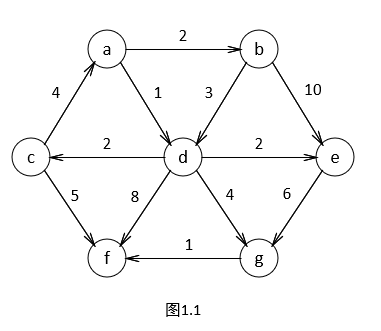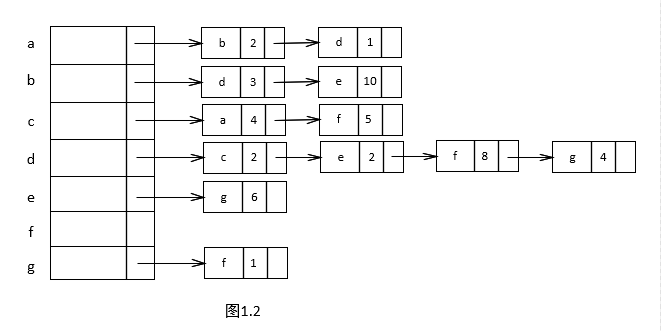#### 实现步骤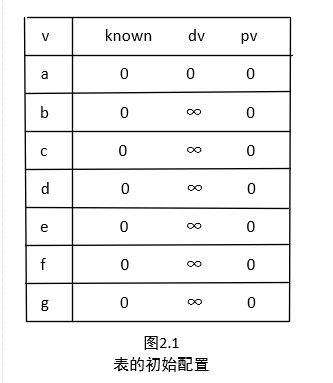Dijkstra算法按阶段进行，在每个阶段，Dijkstra算法选择一个顶点 v ，它在所有未知顶点中具有最小的dv，同时算法声明从 s 到 v 的最短路径是已知的。阶段的其余部分由dw值的更新工作组成。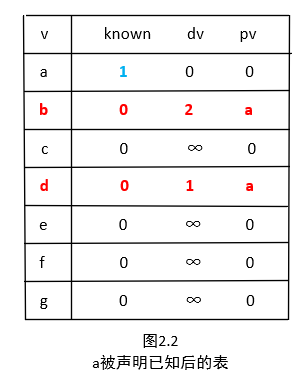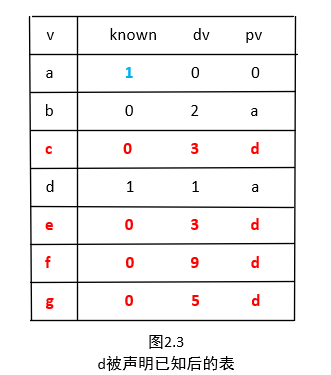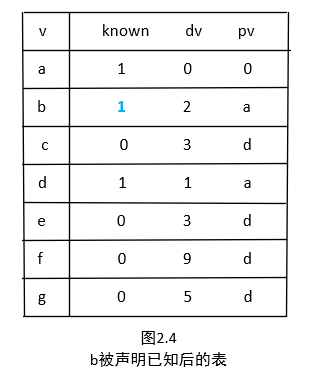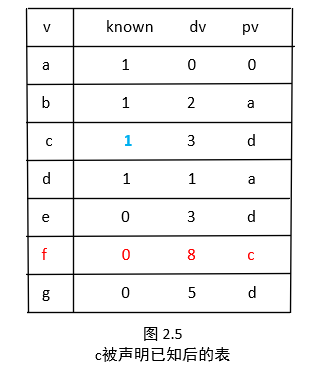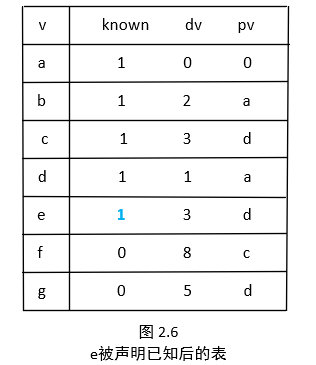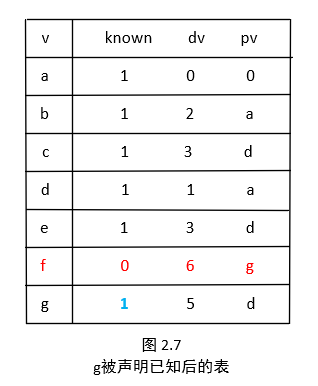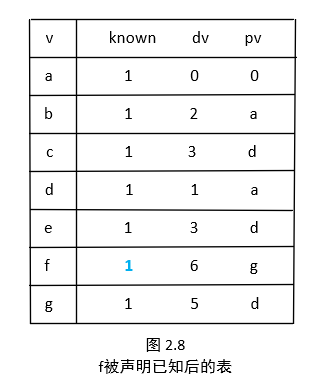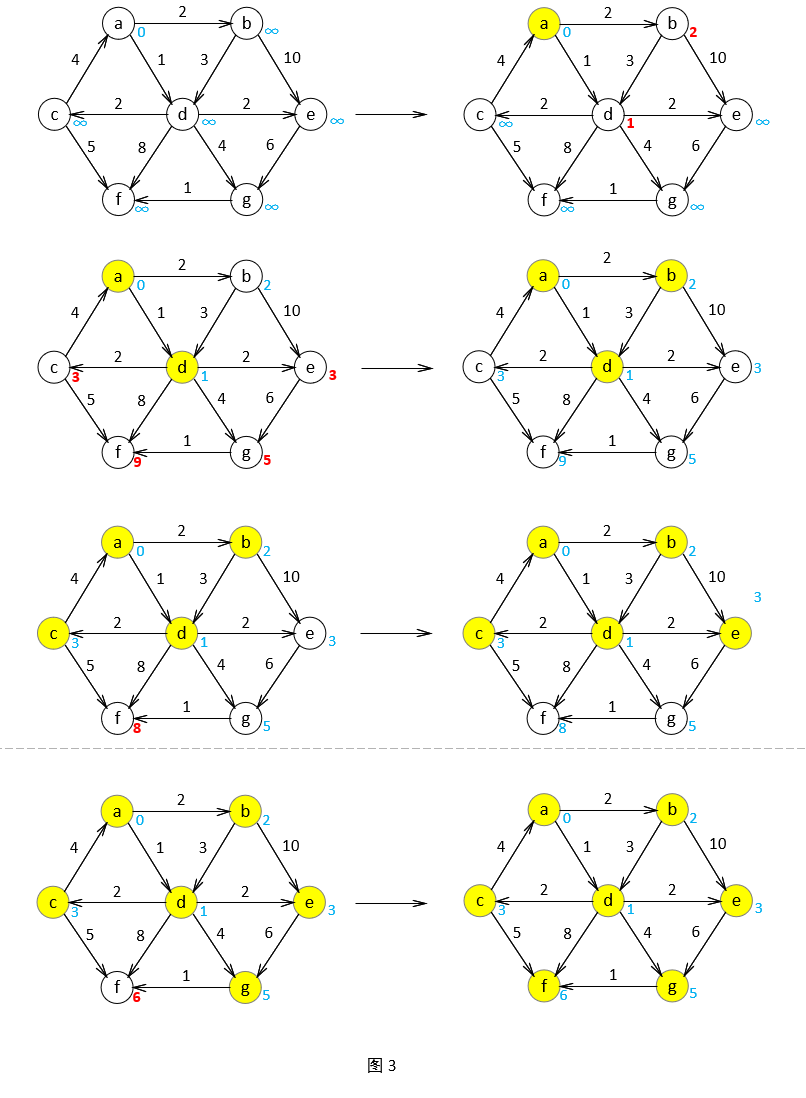#### 代码实现

typedef string vertex;struct Node{    vertex data;    int weight = 0;    Node *next = NULL;    Node(vertex data,int weight, Node *next = NULL)    {        this->data = data;        this->weight = weight;        this->next = next;    }};

/*表  不考虑负边*/const int INFINITE = -1;  //距离 定义无限大struct Box{    bool known = false;    int dv = INFINITE;    vertex path;};

map<vertex, Box*> Dijkstra(vertex v,map<vertex, Node*> graph){    map<vertex, Box*> boxs = initBox(v, graph);    while (1)    {        map<vertex, Box*>::iterator it = boxs.begin();        int min = 99999999;        //key为当前阶段应当标记为已知的顶点        vertex key;        int flag = 0;        while (it != boxs.end())        {            if (it->second->known==false&& it->second->dv!= INFINITE &&it->second->dv < min)            {                min = it->second->dv;    //记录最短路径                key = it->first;        //记录最小路径的key                flag = 1;                //将flag置为1，说明，还有known为false的            }            it++;        }        //所有known都是true ，算法结束        if (flag == 0)        {            return boxs;        }        boxs[key]->known = true;        //将最小的顶点known置为true        //将所有与此顶点相邻接的点进行更新        Node* n = graph[key];        while (n != NULL)        {            //如果此顶点是已知的，就不虚要再处理了            if (boxs[n->data]->known == true)            {                n = n->next;                continue;            }            //此顶点计算出来到it2的路径            int d_new = boxs[key]->dv + n->weight;            //原本it2的路径长度            int d_old = boxs[n->data]->dv;            //需要更新            if (d_old == INFINITE || d_new < d_old)            {                boxs[n->data]->dv = d_new;                boxs[n->data]->path = key;            }            n = n->next;        }    }}

string printp(vertex p, map<vertex, Box*> boxs){    if (boxs[p]->dv == 0)    {        return p+"";    }    return printp(boxs[p]->path, boxs) + "->" + p;}//输出算法的结果map<vertex, string> printfDijkstra(map<vertex, Box*> boxs){    //用来存放输出的内容    map<vertex, string> outs;    vertex aim;    //找到起点    map<vertex, Box*>::iterator it = boxs.begin();    while (it != boxs.end())    {        if (boxs[it->first]->dv == 0) {            aim = it->first;            break;        }        it++;    }    it = boxs.begin();    while (it != boxs.end())    {        if (it->first != aim)        {            string path = printp(boxs[it->first]->path, boxs) + "->" + it->first;            outs.insert(pair<vertex, string>(it->first, path));        }        it++;    }    //outs已经存放好所有路径了    //打印数据    it = boxs.begin();    while (it != boxs.end())    {        if (it->first != aim)        {            cout << it->first + "\t最短路径：" + to_string(boxs[it->first]->dv) + "\t路径：" + outs[it->first] << endl;        }        it++;    }    return outs;}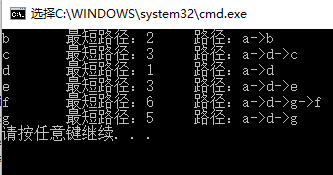## 评论